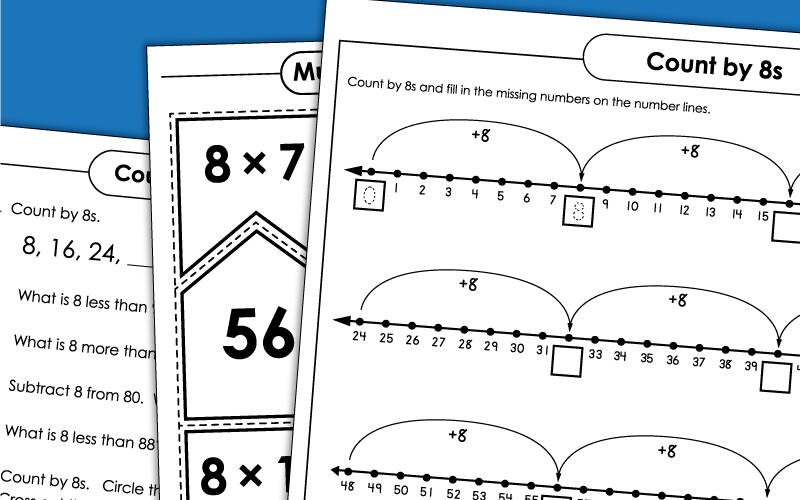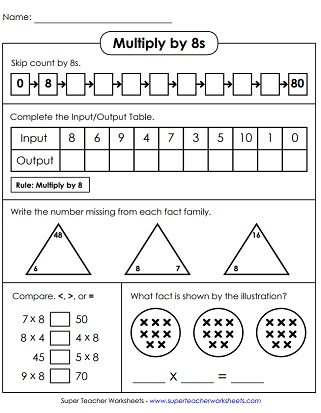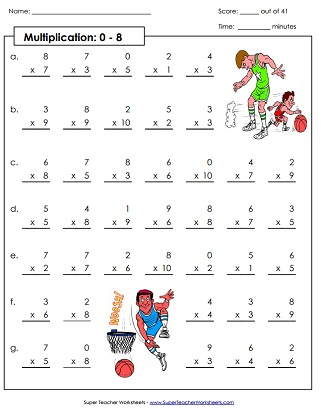# Basic Facts: Multiplying by 8s

On this page, you'll find a series of worksheets for helping kids learn their basic facts up to 8x10. We also have some activities that focus specifically on the 8s.## Multiplication by8s Only

First, students skip count by 8s. Then they complete an input/output box. After that, they compare numbers using the symbols <, >, and =. Then they write a multiplication fact to go along with the illustration.
This math mystery picture has a summery beach scene. Students solve the math problems and write the answers on the page. Then color each space according to the key to see the mystery picture.
Practice multiplying by 8 with this set of task cards. They can be used for activities such as peer practice, scavenger hunts, games, and more.
Place these puzzle pieces on a table or floor. Have small groups match up the multiplication facts with the correct answers.
Students will multiply by 8s in order to fill out all the bubbles in the multiplication caterpillar in this printable math activity.
Students can create an origami toy that can be used to practice basic multiplication facts with factors of eight.
Practice multiplication facts with 8 as a factor. Sort cards into 2 groups on the sorting mat: facts I have mastered and facts I am still practicing.
Print this ladybug craft on heavy card stock. Cut out the picture and the number sliders. Place the slider through the lady bug to see math facts. Students can read facts and say the answer.

## Skip Counting by 8s

Can you students answer these word problems and critical thinking questions? In order to do so, they'll need to know how to skip count by 8s up to 80.
Count by 8s and fill in the missing numbers in the number lines on this skip-counting worksheet.
Skip count from 0 to 224 by 8s. This page has robot-themed graphics.
Write the numbers on the penguin bellies as you skip count. Numbers as high as 192.
This worksheet is very similar to the sheet above, except that you'll write the numbers on the balloons instead of penguins.

## All Facts0s through 8s

Students answer 41 basic fact questions with factors of 0, 1, 2, 3, 4, 5, 6, 7, and 8. Facts through 10s, up to 8x10.
Practice multiplying by 0s, 1s, 2s, 3s, 4s, 5s, 6s, 7s, and 8s. This drill sheet has 39 problems. This version includes 11s and 12s, up to 8x12.
Assess your students' knowledge of basic facts for the 0s, 1s, 2s, 3s, 4s, 5s, 6s, 7s, and 8s times tables. Facts through 12, up to 8x12.
Multiplication Basic Facts: 0-10

Here you'll find lots more multiplication worksheets, games, and activities for all basic facts up to 10x10.

Multiplication/Division
Fact Families

This page has lots of worksheets with multiplication and division fact families.

Multiplication by 3s

Practice multiplying by the number 3. Includes mystery pictures, skip counting lessons, and more.

Multiplication by 4s

These worksheets focus specifically on the 4s times tables.

Multiplication by 5s

Here are the activities for learning multiplication facts from 5x0 up to 5x12.

Multiplication by 6s

Multiplication by 7s

Basic facts with factors of 7 are the focus on these printable learning resources.

Multiplication by 9s

## Sample Worksheet ImagesMy Account
Site Information